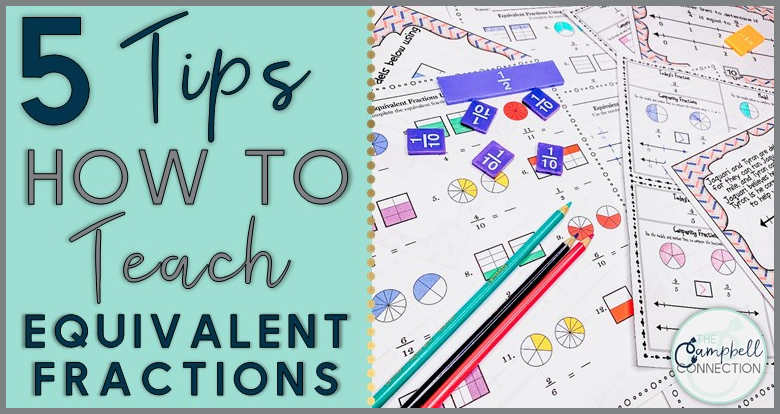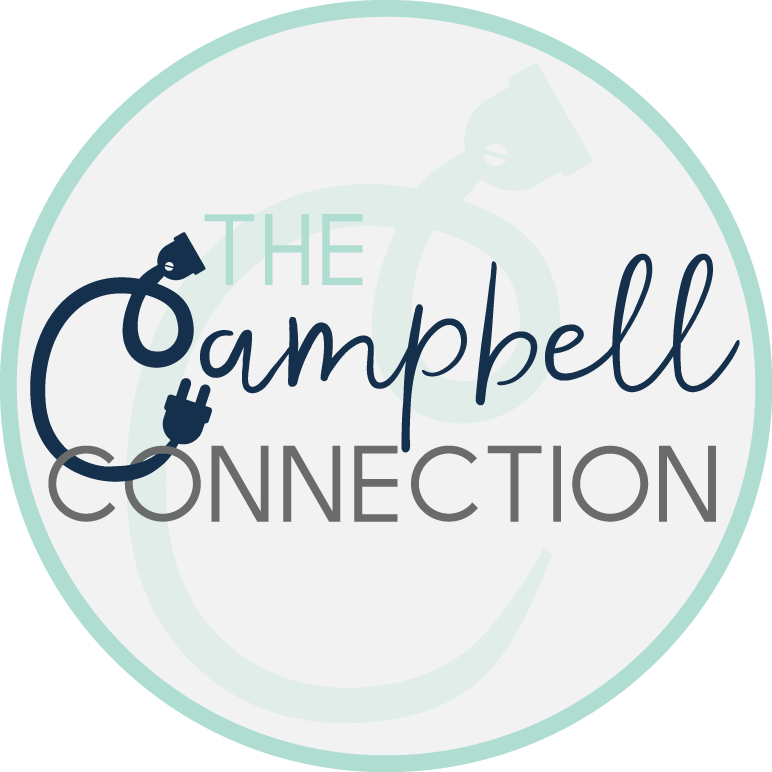connect# 5 Tips for How to Teach Equivalent Fractions

## 5 Tips for How to Teach Equivalent Fractions

How to teach equivalent fractions is the question on every teacher’s mind! It is not a topic for the faint of heart. The definition of equivalent fractions is multiple fractions that have different numerators and denominators but equal the same part of a whole. A few equivalent fractions examples are 2/4 = 1/2, 3/4 = 6/8, 1/3 = 2/6. There are many different strategies and techniques on how to teach equivalent fractions to 3rd graders , but I am here to tell you, the tried and true ones, whether you are more of an equivalent fractions worksheet or equivalent fractions games kind of teacher. I’ve got you covered! Basically, here is the best way to teach equivalent fractions. I am going to tell you all of my secrets and then give you some resources to make your life easier (including a free guide). Let’s work smarter, not harder, together and learn how to teach equivalent fractions!

## How to Teach Equivalent Fractions to 3rd Graders

I am sure you have heard this a thousand times–I take that back, more like a million. The only way your students are going to master equivalent fractions is through using fraction models. Use all the manipulatives, fraction bars and fraction circles. Use them all day every day! Come up with an easy procedure for getting your fraction models passed out to your students. When you are teaching you should be modeling how to use fraction models and your students should be following along with their own set. Basically, fraction models should be very easily accessible and using them should be second nature to your students.

## Equivalent Fractions on a Number Line

Using a number line when teaching equivalent fractions has become more common with the Common Core State Standards. If you do not use this strategy when teaching equivalent fractions, start now! Teach your students how to draw a fractions number line and make them do it over and over again. It will be painful at first, trust me; but that is ok! Learning is supposed to be a little messy. Remember what I said earlier about fraction models? Use fraction bars while teaching students how to create a number line. It will really help! If that is not enough, I have an equivalent fractions number line activity that scaffolds the learning for your students. This is one of the key elements in equivalent fractions how-to!

## Fun Way to Teach Equivalent Fractions

Ok, just a minute. How is food going to help me teach equivalent fractions you ask? Well, let’s be honest. Equivalent fractions are, well, boring. How can you teach them in a way your students will be engaged and excited to learn? Use food! Get some Hershey’s bars and make equivalent fractions with them! This is a fun equivalent fractions activity and students will thank you.

## Using Math Games

My last tip is to use games when teaching equivalent fractions. There are so many equivalent fractions games out there. Task cards are an easy activity to make a game out of; math games are high on every teachers list. Bingo in an oldie but a goodie. What student wouldn’t have fun playing equivalent fraction bingo. They are fun and engaging for students. Plus, they make an easy center for workshop. I am going to be creating an equivalent fractions bingo game soon! Check back for an update on that.

Well, there you go. All of my equivalent fractions how to strategies in one place! Want more ideas on this topic? Click here for a post on Equivalent Fractions Games. Want to get more ideas? Click here to read about equivalent fractions games.

Here is the part you have been waiting for!

Still want more? Click here for my guide on the 5 Equivalent Fractions Hacks.## Hey there!

I’M MELISSA!

I design curriculum and am a self proclaimed tech nerd! I love designing resources to make teachers’ lives easier. In addition, I blog all things education. Let’s get to know each other and connect!

## Search